# Connectedness is product-closed

This article gives the statement, and possibly proof, of a topological space property (i.e., connected space) satisfying a topological space metaproperty (i.e., product-closed property of topological spaces)
View all topological space metaproperty satisfactions | View all topological space metaproperty dissatisfactions
Get more facts about connected space |Get facts that use property satisfaction of connected space | Get facts that use property satisfaction of connected space|Get more facts about product-closed property of topological spaces

## Statement

Suppose$X_i, i \in I$ is a family of nonempty topological spaces, all of which are connected spaces. Then, the product space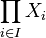$\prod_{i \in I} X_i$, endowed with the product topology, is also a connected space.

## Proof

### Proof outline

The key fact that we use in the proof is that for fixed values of all the other coordinates, the inclusion of any one factor in the product is a continuous map. Hence, every slice is a connected subset.

Now any partition of the whole space into disjoint open subsets must partition each slice into disjoint open subsets; but since each slice is connected, each slice must lie in one of the parts. This allows us to show that if two points differ in only finitely many coordinates, then they must lie in the same open subset of the partition.

Finally, we use the fact that any open set must contain a basis open set; the basis open set allows us to alter the remaining cofinitely many coordinates. Note that it is this part that crucially uses the definition of product topology, and it is the analogous step to this that would fail for the box topology.

### Proof details

Given: An indexing set$I$, a family$(X_i)_{i \in I}$ of nonempty topological spaces.$X$ is the product of these, with the product topology

To prove:$X$ is a connected space. In other words,$X$ cannot be expressed as a disjoint union of two nonempty open subsets$U$ and$V$

ASSUMPTION: Suppose$X$ is a disjoint union of two nonempty open subsets$U$ and$V$.
1 Choose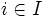$i \in I$ and fix the values of all coordinates except the$i^{th}$ coordinate. Consider the inclusion from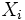$X_i$ to$X$ that sends a point of$X_i$ to the tuple where all other coordinates are the pre-determined ones. This is a subspace embedding and in particular, the image of$X_i$ is a connected subset in$X$.
2 If two elements of$X$ differ in only one coordinate, they are in the same connected component. Step (1) Step-direct -- the image of the corresponding$X_i$ is a connected subset containing both.
3 If two elements of$X$ differ in only finitely many coordinates, they are in the same connected component. Step (2) If two elements differ in finitely many coordinates, we can construct a sequence of elements going from one to the other where adjacent members of the sequence differ in only one coordinate. Any two adjacent members of the sequence are in the same connected component, and thus, all members of the sequence are in the same connected component.
4 If two points of$X$ differ in only finitely many coordinates, they must either both lie in$U$ or both lie in$V$. Assumption Step (3) By Step (3), any two points differing in only finitely many coordinates lie in the same connected component, say$C$. However, if one lies in$U$ and the other lies in$V$, then$C$ itself is a union of non-empty disjoint open subsets of itself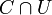$C \cap U$ and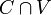$C \cap V$, making it disconnected. Thus, they must both lie in the same piece.
5 Since$U$ is open and non-empty, it contains a basis element -- say, an open subset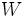$W$ given by$\prod_{i \in I} W_i$ where all the$W_i$ are non-empty and only finitely many of the$W_i$ are not the whole space. Let's call these finite ones$i_1, i_2, \dots, i_n$. definition of product topology
6 For any point of$X$, we can thus find a point of$W$ (and hence of$U$) that differs from it in at most the$n$ coordinates found in Step (5). Step (5) Simply replace the$n$ coordinates by coordinates of elements of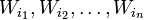$W_{i_1}, W_{i_2}, \dots, W_{i_n}$ and leave the remaining coordinates as they are.
7 Any point of$X$ is in$U$, completing the contradiction to the assumption that$V$ is nonempty. Steps (4), (6) By Step (6), we can, for any point in$X$, find an element of$U$ that differs from it in finitely many coordinates. Thus, by Step (4), the point in$X$ that we start with must also be in$U$.